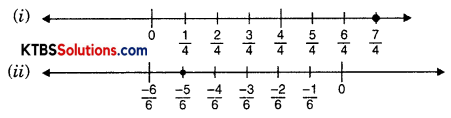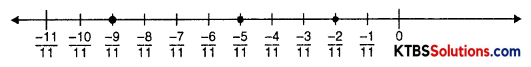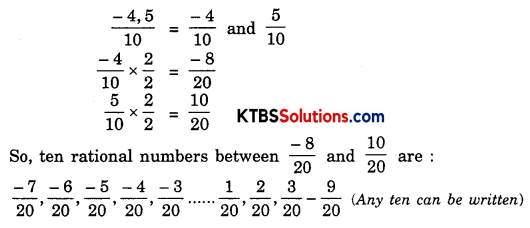# KSEEB Solutions for Class 8 Maths Chapter 1 Rational Numbers Ex 1.2

You can Download KSEEB Solutions for Class 8 Maths Chapter 1 Rational Numbers Ex 1.2 Questions and Answers helps you to revise the complete syllabus.

## KSEEB Solutions for Class 8 Maths Chapter 1 Rational Numbers Ex 1.2

Question 1.
Represent these numbers on the number line.
(i) $$\frac{7}{4}$$
(ii) $$\frac{-5}{6}$$
Solution:Question 2.
Represent $$\frac{-2}{11}, \frac{-5}{11}, \frac{-9}{11}$$ on the number line.
Solution:Question 3.
Write five rational numbers which are smaller than 2.
Solution:
Five rational numbers smaller than 2 are 1, 0, $$\frac{-1}{2}$$, -1, -2 (In this way infinite numbers can be written)Question 4.
Find ten rational numbers between $$\frac{-2}{5}$$ and $$\frac{1}{2}$$
Solution:
$$\frac{-2}{5}$$ and $$\frac{1}{2}$$Question 5.
Find five rational numbers between:
(i) $$\frac{2}{3}$$ and $$\frac{4}{5}$$
(ii) $$\frac{-3}{2}$$ and $$\frac{5}{3}$$
(iii) $$\frac{1}{4}$$ and $$\frac{1}{2}$$
Solution:
(i) $$\frac{2}{3}=\frac{2}{3} \times \frac{5}{5}=\frac{10}{15}=\frac{30}{45}$$
$$\frac{4}{5}=\frac{4}{5} \times \frac{3}{3}=\frac{12}{15}=\frac{36}{45}$$
So, five rational numbers between $$\frac{30}{45}$$ and $$\frac{36}{45}$$ are $$\frac{31}{45}, \frac{32}{45}, \frac{33}{45}, \frac{34}{45}, \frac{35}{45}$$

(ii) $$\frac{-3}{2}=\frac{-3 \times 3}{2 \times 3}=\frac{-9}{6}$$
$$\frac{5}{3}=\frac{5}{3} \times \frac{2}{2}=\frac{10}{6}$$
So, five rational numbers between $$\frac{-9}{6}$$ and $$\frac{10}{6}$$ are $$\frac{-8}{6}, \frac{-7}{6}, \frac{-6}{6}, \frac{1}{6}, \frac{2}{6}, \ldots \frac{9}{6}$$ (Any five can be written)

(iii) $$\frac{1}{4}$$ and $$\frac{1}{2}$$
$$\frac{1}{4}=\frac{1}{4} \times \frac{2}{2}=\frac{2}{8} \times \frac{5}{5}=\frac{10}{40}$$
$$\frac{1}{2}=\frac{1}{2} \times \frac{4}{4}=\frac{4}{8} \times \frac{5}{5}=\frac{20}{40}$$
So, five rational numbers between $$\frac{10}{40}$$ and $$\frac{20}{40}$$ are $$\frac{11}{40}, \frac{12}{40}, \frac{13}{40}, \frac{14}{40}, \frac{15}{40} \ldots \ldots \ldots$$

Question 6.
Write five rational numbers greater than -2.
Solution:
Five rational numbers greater than -2 are -1, 0, 1, 2, 3, 4 (or many more)Question 7.
Find ten rational numbers between $$\frac{3}{5}$$ and $$\frac{3}{4}$$.
Solution:
$$\frac{3}{5}$$ and $$\frac{3}{4}$$
$$\frac{3}{5} \times \frac{4}{4}=\frac{12}{20}=\frac{12}{20} \times \frac{5}{5}=\frac{60}{100}$$
$$\frac{3}{4} \times \frac{5}{5}=\frac{15}{20}=\frac{15}{20} \times \frac{5}{5}=\frac{75}{100}$$
ten rational numbers between $$\frac{60}{100}$$ and $$\frac{75}{100}$$ are $$\frac{61}{100}, \frac{62}{100}, \frac{63}{100} \ldots \ldots . . \frac{74}{100}$$ (Out of these any ten can be taken)